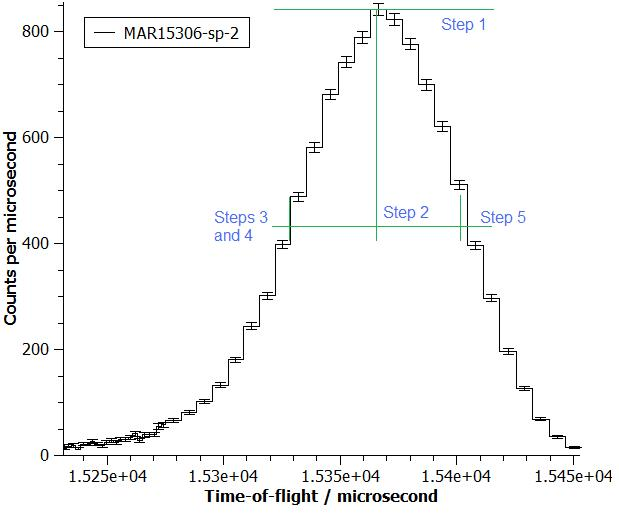$$\renewcommand\AA{\unicode{x212B}}$$

# GetEi v1¶

## Summary¶

Calculates the kinetic energy of neutrons leaving the source based on the time it takes for them to travel between two monitors.

## Properties¶

Name

Direction

Type

Default

Description

InputWorkspace

Input

MatrixWorkspace

Mandatory

The X units of this workspace must be time of flight with times in micro-seconds

Monitor1Spec

Input

number

Mandatory

The spectrum number of the output of the first monitor, e.g. MAPS 41474, MARI 2, MERLIN 69634

Monitor2Spec

Input

number

Mandatory

The spectrum number of the output of the second monitor e.g. MAPS 41475, MARI 3, MERLIN 69638

EnergyEstimate

Input

number

Mandatory

An approximate value for the typical incident energy, energy of neutrons leaving the source (meV)

IncidentEnergy

Output

number

FirstMonitorPeak

Output

number

## Description¶

Uses $$E=\frac{1}{2}mv^2$$ to calculate the energy of neutrons leaving the source. The velocity is calculated from the time it takes for the neutron pulse to travel between the two monitors whose spectra were specified.

An initial energy guess is required for the algorithm to find the correct peak. The analysis will be done on the highest peak that is within 8% of the estimated TOF given by the estimate.

Not all neutrons arrive at the monitors at the same time because their kinetic energies, and therefore velocities, are all different. The time of arrival of the neutron pulse is taken to be the mean of the two half peak height locations. The half height points are found as follows:

1. the peak height is the largest number of counts above the background in any bin in the window

2. the half height is half the above number

3. examine bins to the left of the bin with the highest number of counts looking for a bin with less than half that number above background

4. interpolate between this point bin and the one immediately previous to find the first half height location

5. repeat the steps 3 and 4 looking to the right of the highest point to get the second half height point

6. the mean of the X-values of the two half height points is the TOF arrival time of the neutrons

The above process is illustrated on a peak is shown below in the image belowThe distances between the monitors are read from the instrument definition file. It is assumed that the source and the monitors all lie on one line and that the monitors have the same delay time.

Warning

This version is an older algorithm and is no longer in current use, although it is not deprecated.

Categories: AlgorithmIndex | Inelastic\Ei